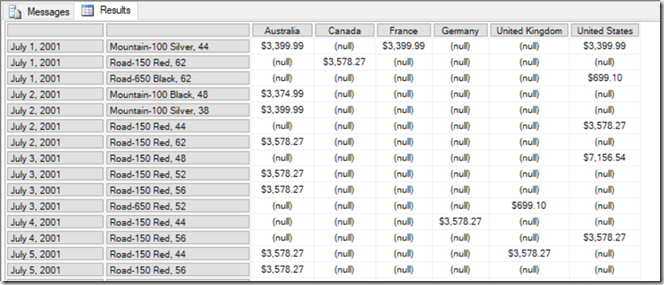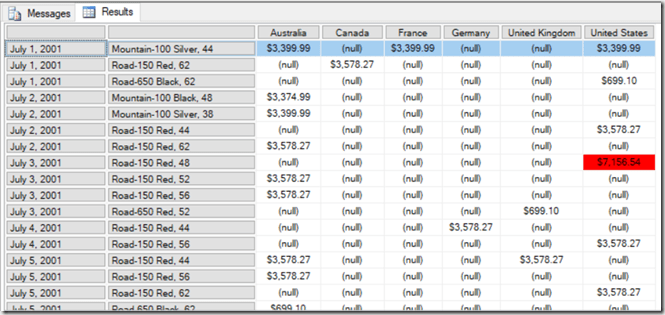# Obscure MDX Month: Optimising MDX That Uses The RGB() Function

In the first blog post in this series a few weeks ago I mentioned that calling Excel and VBA functions from MDX came with a query performance penalty. In this post I’ll give you an illustration of this using the VBA function that I suspect is most frequently called in MDX: the RGB() function.

Take the following MDX query as a baseline:

```WITH
MEMBER MEASURES.TEST AS
[Measures].[Internet Sales Amount]
SELECT {[Customer].[Country].[Country].MEMBERS} ON 0,
NON EMPTY
[Date].[Date].[Date].MEMBERS
*
[Product].[Product].[Product].MEMBERS
ON 1
FROM
WHERE(MEASURES.TEST)
CELL PROPERTIES
VALUE,
FORMATTED_VALUE,
BACK_COLOR
```

It returns Countries on columns and all non empty combinations of Date and Product on rows, and the calculated measure returns the value of the Internet Sales Amount measure:On a SE engine cache it runs in 2.5 seconds on my laptop. With a BACK_COLOR property added to the calculated measure that uses the RGB() function to return the code for red if the measure value is greater than \$5000, query performance is a lot worse: it goes up to 6.5 seconds on a warm SE cache.

```WITH
MEMBER MEASURES.TEST AS
[Measures].[Internet Sales Amount]
,BACK_COLOR=
IIF([Measures].[Internet Sales Amount]>5000,
RGB(255,0,0),
RGB(255,255,255))
SELECT {[Customer].[Country].[Country].MEMBERS} ON 0,
NON EMPTY
[Date].[Date].[Date].MEMBERS
*
[Product].[Product].[Product].MEMBERS
ON 1
FROM
WHERE(MEASURES.TEST)
CELL PROPERTIES VALUE, FORMATTED_VALUE, BACK_COLOR
```That’s a big increase just to do some cell highlighting! However in this case the RGB() function can only return two possible integer values, so if you replace the RGB() function with the integers it returns, like so:

```WITH
MEMBER MEASURES.TEST AS
[Measures].[Internet Sales Amount]
,BACK_COLOR=
IIF([Measures].[Internet Sales Amount]>5000,
255,
16777215)
SELECT {[Customer].[Country].[Country].MEMBERS} ON 0,
NON EMPTY
[Date].[Date].[Date].MEMBERS
*
[Product].[Product].[Product].MEMBERS
ON 1
FROM
WHERE(MEASURES.TEST)
CELL PROPERTIES VALUE, FORMATTED_VALUE, BACK_COLOR
```

…then the query returns in around 3.5 seconds. The last thing to remember is that IIF() statements can perform better if one branch returns null, and in this case we can replace the integer value 16777215 that gives the white background with a null and get the same result:

```WITH
MEMBER MEASURES.TEST AS
[Measures].[Internet Sales Amount]
,BACK_COLOR=
IIF([Measures].[Internet Sales Amount]>5000,
255,
16777215)
SELECT {[Customer].[Country].[Country].MEMBERS} ON 0,
NON EMPTY
[Date].[Date].[Date].MEMBERS
*
[Product].[Product].[Product].MEMBERS
ON 1
FROM